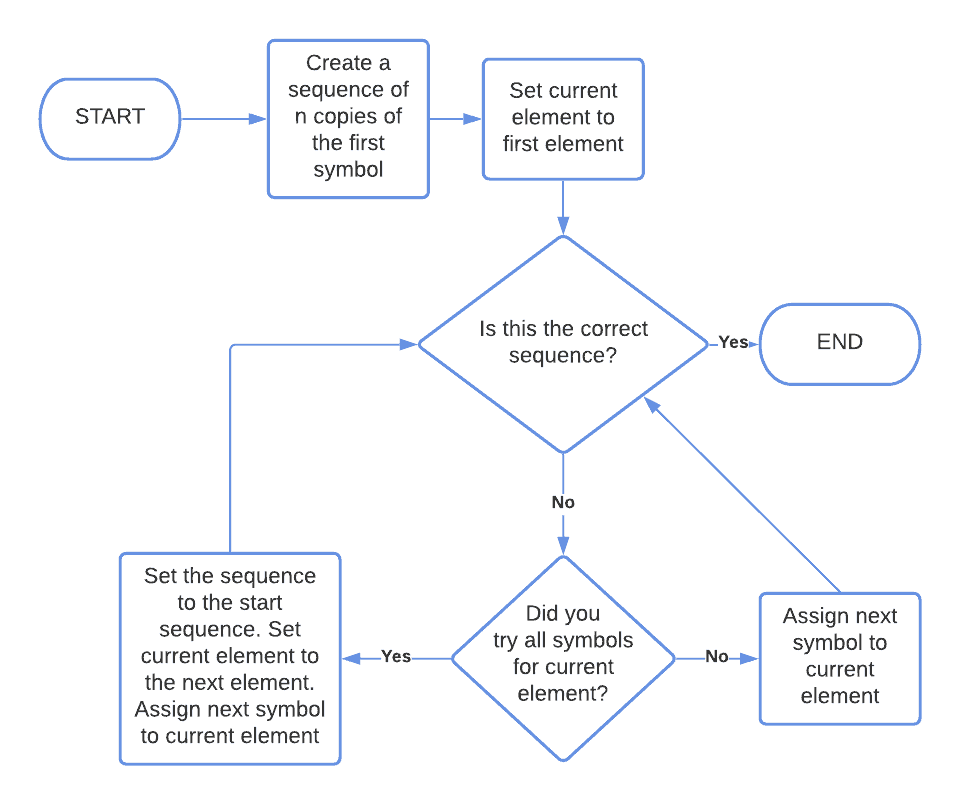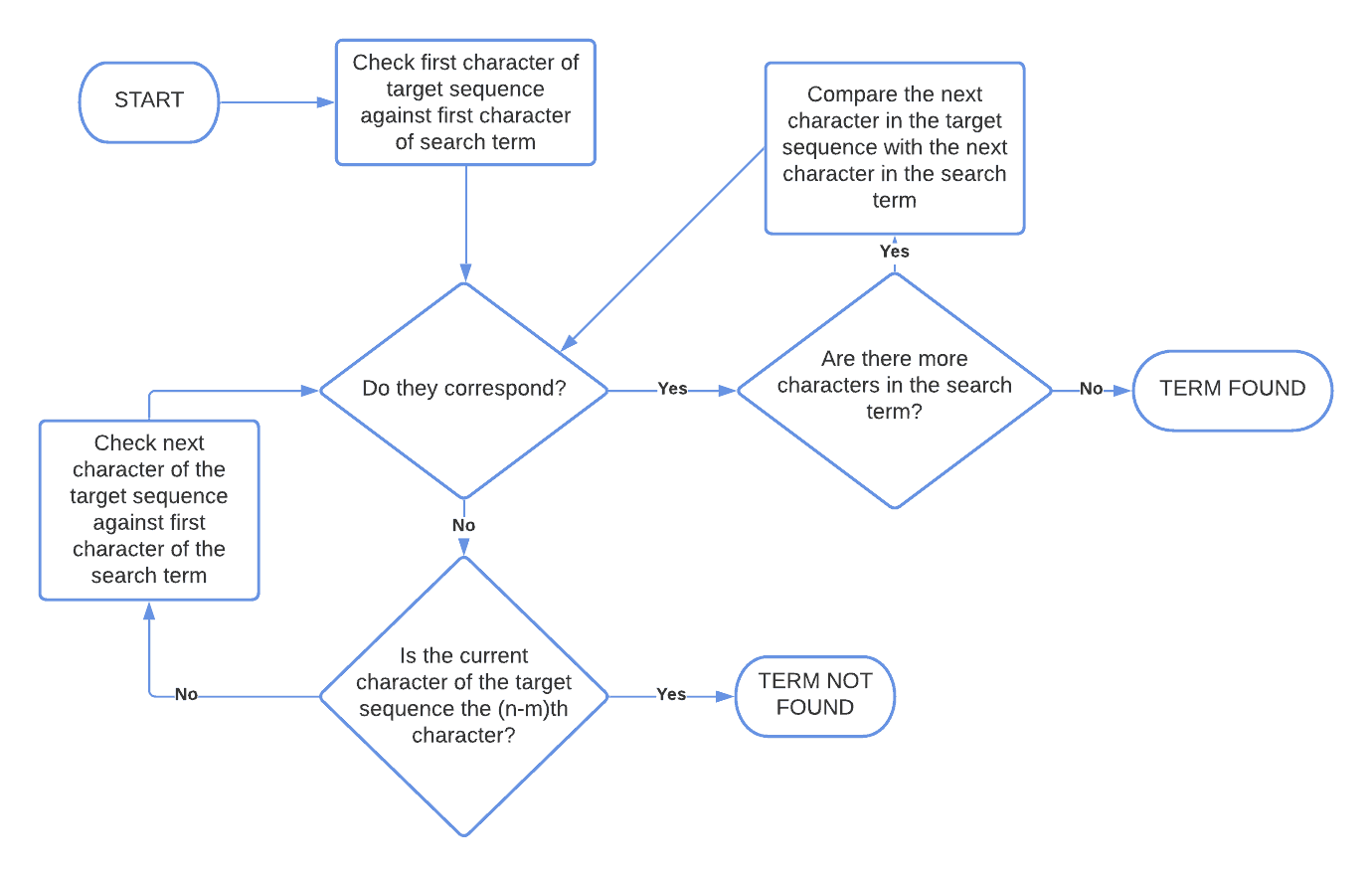## 1. Overview

In this tutorial, we’ll study the brute-force algorithm and its characteristics.

We’ll first define the article in its more general formulation, in terms of combinatorics. Then, we’ll see its application in a string search.

At the end of this tutorial, we’ll know how a brute-force search works and how to implement it in practice.

## 2. A Force That’s Brute

Brute-force is an algorithm for exhausting a problem by testing all of its possible solutions or, in terms of strings searches, for finding a substring by checking all of its possible positions. It’s commonly studied in the sector of network security, because of the frequent encountering of brute-force attempts for unauthorized authentication.

The reason for its name lies in the fact that the algorithm attempts all possible solutions, in order, and without any particular preference. In this sense, the algorithm embeds no knowledge of the problem, which makes it therefore either dull or brute.

Here, we first analyze the brute-force algorithm for the more general problem of sequence matching. Then, we can derive its simplified version for substring matching in strings.

### 2.1. Brute-Force for Known-Length Sequences

A brute-force search is possible if we know thesymbolsthat a target sequence can contain. If we also know the lengthof that sequence, we then proceed in this order:

1. First, we try the sequencecomprised ofrepetitions of2. Then, if the sequence fails, we change the first element ofto3. If this also fails, we change sequentially the first element ofto all possible symbols in, in order
4. If this fails too, we change the second element oftoand repeat from the start
5. Finally, we sequentially test all possible symbols inas the second element ofand, if that fails, we continue with all the other elements ofuntil we find a solution

This is the flowchart of the algorithm:### 2.2. Computational Time

The algorithm requires the testing of allpossible combinations in the worst-case scenario. For this reason, its computational time is, forandasymptotically large.

Notice that this grows very quickly, as the parametersandincrease. As an example, if the sequence has length 2 and 2 possible symbols, the algorithm then tests a maximum ofpossible combinations:If, however, the sequence has lengthand uses as symbols thecharacters of the alphabet, as some basic passwords may, we then havepossible combinations:Finally, if the symbol set is the 128 characters of ASCII, and the target sequence has a length of 10, then the number of possible combinationsis close to. If we performedattempts per second, it would take us aroundyears to test all possible combinations.

There’s also a great degree of variability in the actual computational time. Because we don’t have any reason to believe that the target sequence is located in a particular section of the search space, the expected time for completion is. The best-case, instead, is, if the first value attempted is the target value.

### 2.3. Sequences of Unknown Length

If we don’t know the lengthof the target sequence, but we know it’s finite, we need to test all possible lengths until we find the right one. This means that we first test the algorithm against a sequence of length one, which we can do intime.

The second iteration requirestime, which is significantly larger than. Therefore, forasymptotically large, the first two iterations requiretime.

If we repeat this argument, forandasymptotically large the computational time remains, as was the case for sequences of fixed length.

The algorithm for brute-force search in a string is based upon the same underlying principle as the previous one. In this case, though, we’re searching whether a string of lengthcontains a substring of length. If it does, we want to know its position in the string.

We’ll see an example of usage first, and then its formalization.

### 3.1. Searching for Truth

In this example, we’re searching for the word “truth” in Jane Austen’s famous quote:In the first step of the algorithm, we compare the first character of the string with our search term:Because the comparison fails, we shift the comparison to the second element of the target sequence, and to the first character of our search term:Because the comparison succeeds, we can try to test the second element of our search term with the next element of the target sequence:This time we’re not so lucky. We can, however, move forward, and keep repeating the comparisons. We do so until we reach the element with index 8:The sequential comparison of all elements of the search term with all elements of the target sequence, starting from that index, is here successful. This means that we identified the search term as the substring of characters comprised betweenandof the target sequence.

### 3.2. Flowchart of the Algorithm

This is the flowchart of brute-force string search:### 3.3. Computational Time

The algorithm terminates its comparison by identifying the target sequence as the substring comprised between 0 and, in the best case. In the worst case, it finds it betweenandor doesn’t find it at all.

Its time complexity is, and it performs on averagecomparisons between characters.

## 4. Conclusion

In this article, we studied the definition of a brute-force search for combinatorial problems and for fixed-length strings.